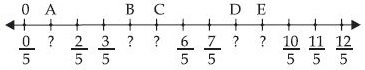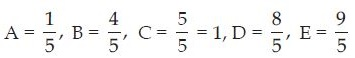# NCERT Solutions for Class 8 Math Chapter 1 - Rational Numbers

##### Question 1:

Fill in the blanks in the following table: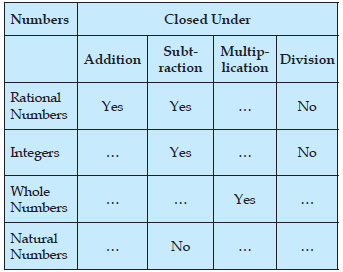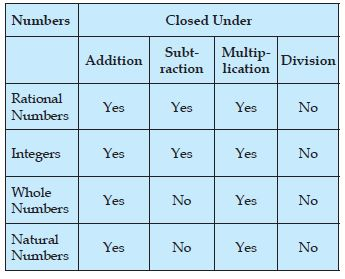##### Question 2:

Complete the following table: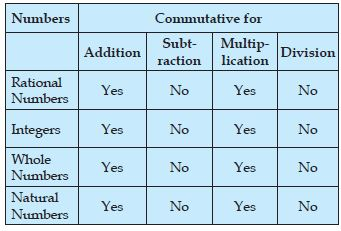##### Question 3:

Complete the following table: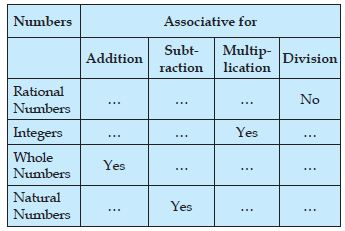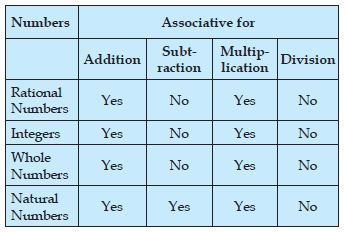##### Question 4:

Find using distributivity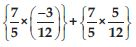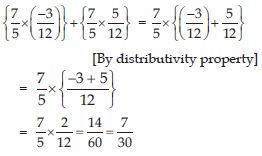##### Question 5:

Find using distributivity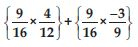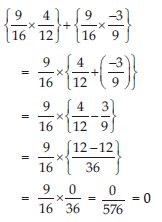##### Question 6:

Using appropriate properties find: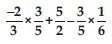##### Question 7:

Using appropriate properties find: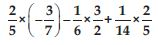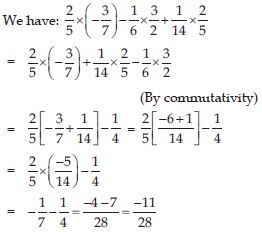##### Question 8:

Write the additive inverse of the following: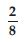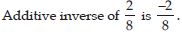##### Question 9:

Write the additive inverse of the following: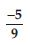##### Question 10:

Write the additive inverse of the following: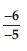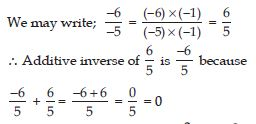##### Question 11:

Write the additive inverse of the following: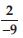##### Question 12:

Write the additive inverse of the following: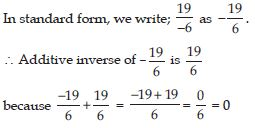##### Question 13:

Verify that: −(−x) = x for: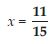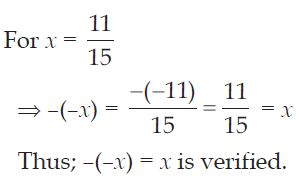##### Question 14:

Verify that: −(−x) = x for: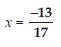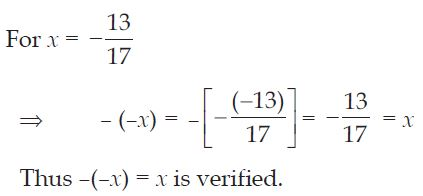##### Question 15:

Find the multiplicative inverse of the following:
–13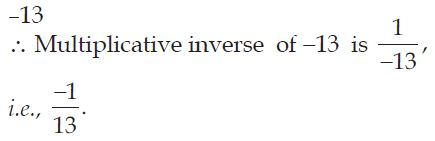##### Question 16:

Find the multiplicative inverse of the following: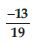##### Question 17:

Find the multiplicative inverse of the following: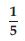##### Question 18:

Find the multiplicative inverse of the following: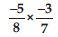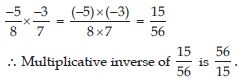##### Question 19:

Find the multiplicative inverse of the following: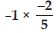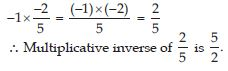##### Question 20:

Find the multiplicative inverse of the following:
–1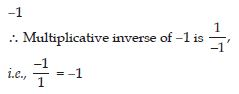##### Question 21:

Name the property under multiplication used of the following: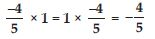Multiplicative identity.

##### Question 22:

Name the property under multiplication used of the following: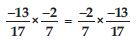Commutative property of multiplication.

##### Question 23:

Name the property under multiplication used of the following: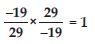Multiplicative inverse.

##### Question 24: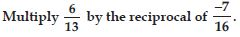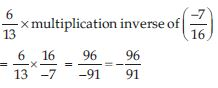##### Question 25:

Tell what property allows you to compute: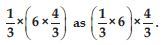Associativity of multiplication.

##### Question 26: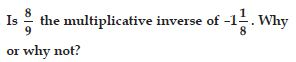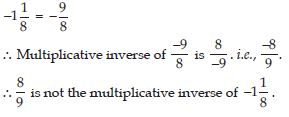##### Question 27: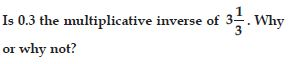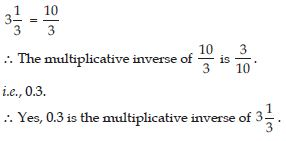##### Question 28:

Write:
(i) The rational number that does not have a reciprocal.
(ii) The rational numbers that are equal to their reciprocals.
(iii) The rational numbers that is equal to their negative.

(i)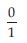(ii) 1 and (–1).
(iii) Zero.

##### Question 29:

Zero has _______________reciprocal.

no

##### Question 30:

The numbers ______________ and ____________ are their own reciprocals.

1 and –1

##### Question 31:

The reciprocal of –5 is ___________ .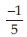##### Question 32:

Reciprocal of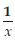, where x $\ne$ 0 is _____________.

x

##### Question 33:

The product of two rational numbers is always a _____________.

rational number

##### Question 34:

The reciprocal of a positive rational number is __________.

positive

##### Question 35:

Represent these numbers on the number line: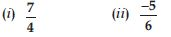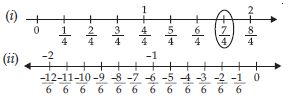##### Question 36: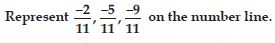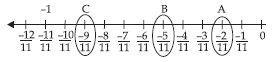##### Question 37:

Write five rational numbers which are smaller than 2.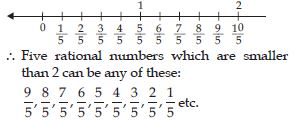##### Question 38: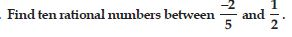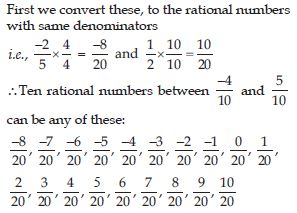##### Question 39:

Find five rational numbers between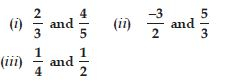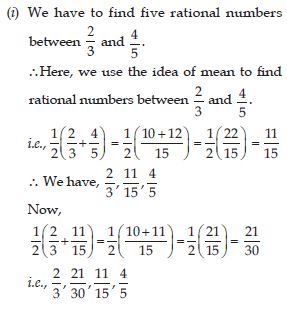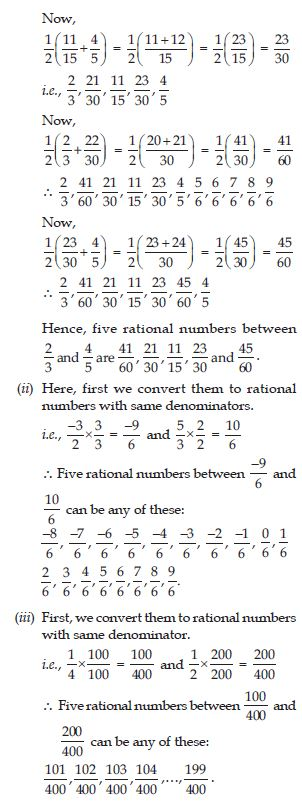##### Question 40:

Write five rational numbers greater than −2.

Five rational numbers greater than −2 are given by : –1, 0, 1, 2, 3, 4, 5 etc.

##### Question 41: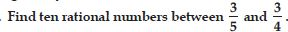First convert them to rational numbers, with the same denominators.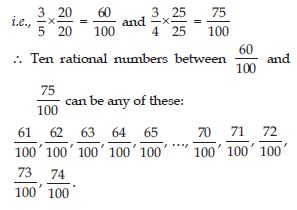##### Question 42:

Write the rational number for each point labelled with a letter.